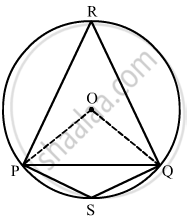Advertisement Remove all ads

# Prove that the Angle in a Segment Shorter than a Semicircle is Greater than a Right Angle. - Mathematics

Short Note

Prove that the angle in a segment shorter than a semicircle is greater than a right angle.

Advertisement Remove all ads

#### Solution$\stackrel\frown{QP} \text{ is a major arc and } \angle PSQ \text{ is the angle formed by it in the alternate segment } .$
$\text{ We know that the angle subtended by an arc at the centre is twice the angle subtended by it at any point of the alternate segment of the circle } .$

=> 2angle "PSQ" = "m"

=> 2angle "PSQ" = 360^circ - "m"

=> 2 angle"PSQ" = 360^circ - 180^circ    ...(because angle "POQ" < 108^circ)

=> 2angle "PSQ" > 180^circ

=> angle "PSQ" > 90^circ

Thus, the angle in a segment shorter than a semi-circle is greater than a right angle.

Is there an error in this question or solution?
Advertisement Remove all ads

#### APPEARS IN

RD Sharma Mathematics for Class 9
Chapter 15 Circles
Exercise 15.5 | Q 27 | Page 104
Advertisement Remove all ads

#### Video TutorialsVIEW ALL 

Advertisement Remove all ads
Share
Notifications

View all notifications

Forgot password?
Course